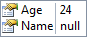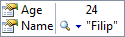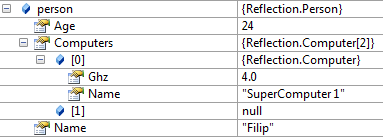# Setting values by using reflection

## Posted by Filip Ekberg on 10 Oct 2011

In all my latest posts regarding reflection all I've done is getting values and invoking methods and this can confuse you into thinking that when using reflection stuff is read only, but it's not. Just as we have `GetValue` we also have `SetValue`.

Consider that we have the following class:

``````class Person
{
public string Name { get; set; }
public int Age { get; set; }
}
``````

Then we've created an instance of it like this:

``````var person = new Person { Age = 24 };
``````

If we inspect the person-object now this is what it will look like:Of course `Name` will be null, we haven't set it to anything yet. In order to set it, we need to get the `Type` object for the Person-class, just like we've done before:

``````Type personType = typeof (Person);
``````

In order to set a value on a property, we must first get the `PropertyInfo` for the property, we actually don't have to get all properties and then use the LINQ-query to get just that property like we've done before.

There's a method called `GetProperty` that takes a string which represents the name of the property that we want to get.

This means that we can write the following to get the `PropertyInfo` for the Name-property:

``````PropertyInfo nameProperty = personType.GetProperty("Name");
``````

Now in order to actually set a value on the Name-property, we call `SetValue`, unlike the `GetValue` method, this one takes three arguments, an extra argument for the actual value that we want to set it to.

So to set the value on the name-property on our person-instance we write the following:

``````nameProperty.SetValue(person, "Filip", null);
``````

The last parameter defines the index of the property where the value should be set, this is used when an object implements the following:

``````public string this[int Index]
``````

So if we inspect the person-object now we should see the following:As you might have noticed it's quite similar to how you retrieve values. Let's look at how we handle arrays, it's pretty similar to what we've just seen, first of all, I added a new object called `Computer` that looks like this:

``````class Computer
{
public string Name { get; set; }
public double Ghz { get; set; }
}
``````

Then I added a property to the Person-class that looks like this:

``````public Computer[] Computers { get; set; }
``````

Now what I want to do is to define that my person has the possibility to get two computers, then I want to assign him a super computer with the following specifications:

``````var superComputer = new Computer { Name = "SuperComputer 1", Ghz = 4.0 };
``````

In order to add something to the Computers property, we must first retrieve it from the Person-type like this:

``````PropertyInfo computersProperty = personType.GetProperty("Computers");
``````

Now I can just assign it a value and I create an instance of an array with two slots:

``````computersProperty.SetValue(person, new Computer, null);
``````

One thing that is worth thinking about here is that we are working with reference types, which means that if I just retrieve the reference to that array, I can manipulate it! Something like this is what I had in mind:

``````var computers = (Computer[])computersProperty.GetValue(person, null);
computers = superComputer;
``````

But I want to make this a bit more interesting, so I am going to use the last parameter on `SetValue`, the indexer. To do this, I need to define how my class will handle indices like this:

``````public Computer this[int Index]
{
get
{
return Computers[Index];
}
set
{
Computers[Index] = value;
}
}
``````

It might not be very logical that you receive a computer when you write `person`, but for the sake of this blog post, I won't confuse you with a lot of changes in the code, so let's get to it! The default name for the index-property is called `Item` and you retrieve it like you've seen before:

``````PropertyInfo indexProperty = personType.GetProperty("Item");
``````

Then we can use this property info to set the value on our person object and passing what index we want to change in the last parameter, like this:

``````indexProperty.SetValue(person, superComputer, new object[] { 0 });
``````

In both this case and the previous case where I just got the reference and worked directly with that, this will be the result when we inspect the person instance: